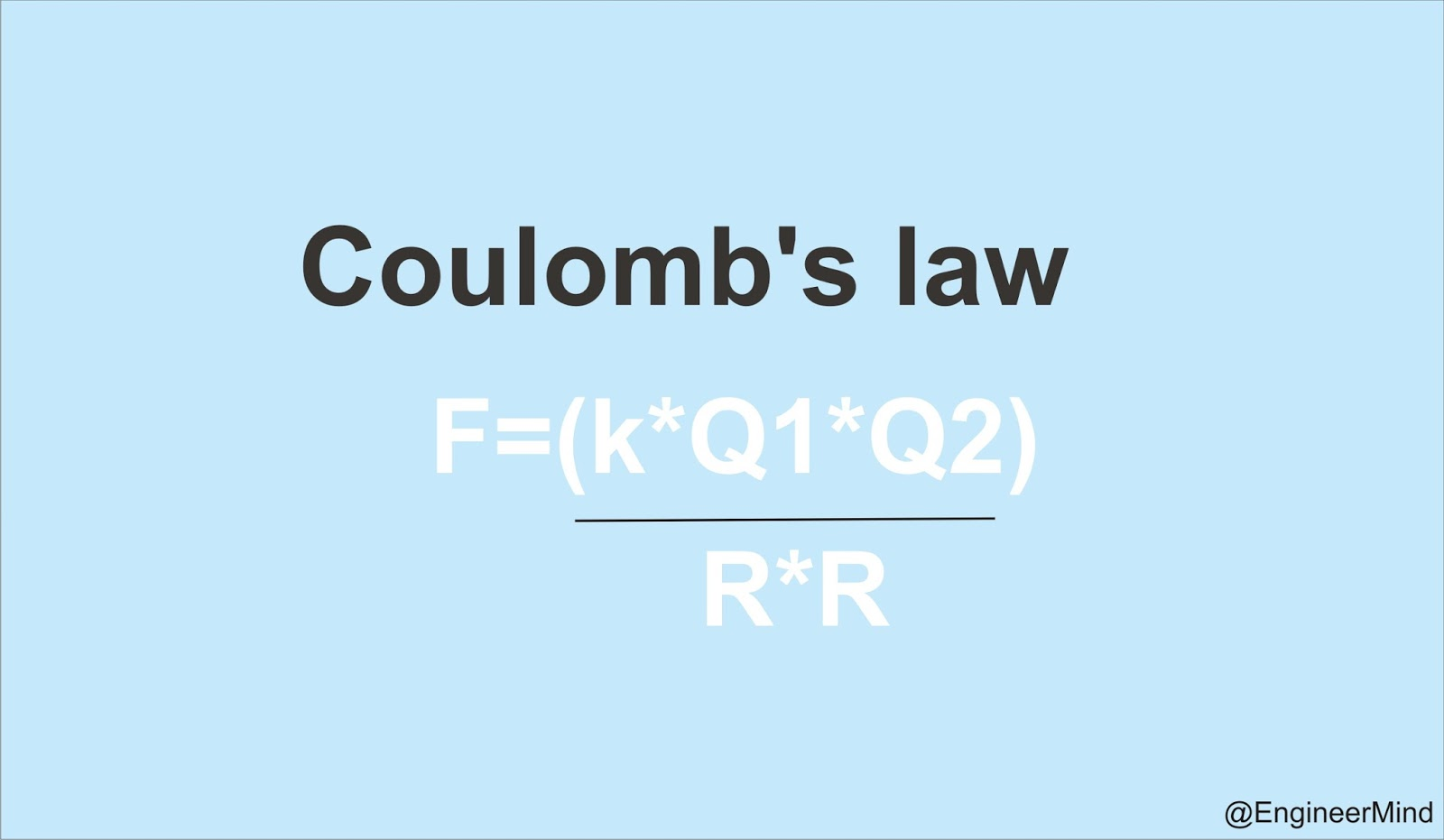# Coulomb’s Law With Example

## How to Find Cutting Length of Bent Up Bars in Slab

Dark Light

### Coulomb’s law :Coulomb’s law calculates the electric force F in newtons (N) between two electric charges q1and q2 in coulombs (C)
with a distance of r in meters (m):F is the force on q1 and q2 measured in newtons (N).
k is Coulomb’s constant k = 8.988×109 N⋅m2/C2
q1 is the first charge in coulombs (C).
q2 is the second charge in coulombs (C).
r is the distance between the 2 charges in meters (m).
When charges q1 and q2 is increased, the force F is increased.
When distance r is increased, the force F is decreased.

### Coulomb’s law example:

Find the force between 2 electric charges of 2×10-5C and 3×10-5C with distance of 40cm between them.
q= 2×10-5C
q= 3×10-5C
= 40cm = 0.4m
F = k×q1×q/ r2
= 8.988×109N⋅m2/C2 × 2×10-5C × 3×10-5C / (0.4m)2
= 37.705N
##### Related Posts## What Happens to Extra Electricity in Power Grids?

There is no extra supply. That would violate a basic principle of grid stability as expressed in what…

## Basic Working of A Thermal Power Station

Almost two third of electricity requirement of the world is fulfilled by thermal power stations. In these power stations, steam is…## Who Invented The Mobile Phone📱?

Have you ever questioned who invented the mobile phone? It is impossible to imagine a world without mobile…

## Procedure Of Acidity Test For Transformer’s Insulating Oil

Importance of Acidity in Insulating Oil Increased acidity of the oil, causes a decrease in resistivity of the…